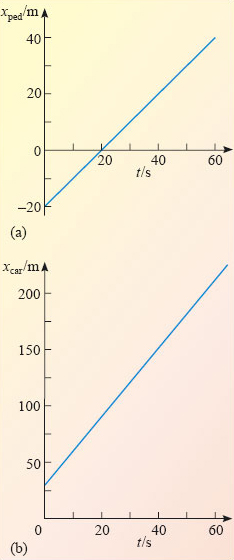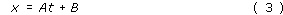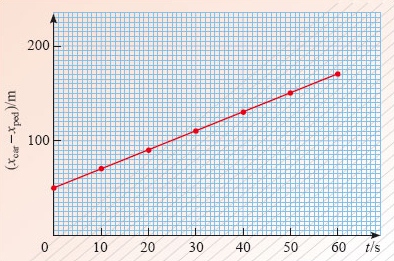Describing motion along a line

This free course is available to start right now. Review the full course description and key learning outcomes and create an account and enrol if you want a free statement of participation.

Free course

# 3 Uniform motion along a line

## 3.1 Describing uniform motion

Uniform motion along a line is the very special kind of motion that occurs when an object moves with unvarying speed in a fixed direction. During a fixed period of time, such as one second, an object in uniform motion will always cover the same distance, no matter when the period begins. This is the kind of motion associated with traffic-free motoring along straight roads, with uninterrupted train journeys along straight tracks, and with unhindered straight and level flying (provided the distances involved are sufficiently small that the curvature of the Earth can be ignored).

Table 4 shows some typical values of position and time for a pedestrian and a car that are both in a state of uniform motion. The corresponding position-time graphs are shown in Figure 12a and b.

### Table 4: Positions for the pedestrian, xped, and the car, xcar, at times t

t/s xped/m xcar/m
0 −20 30
10 −10 60
20 0 90
30 10 120
40 20 150
50 30 180
60 40 210Figure 12: Position-time graphs for the positions of (a) a pedestrian and (b) a car, based on Table 4

As you can see, both are straight-line graphs, as is characteristic of uniform motion. The graphs indicate that in each case the quantities being plotted are related by an equation of the general formwhere A and B are constants. The two parts of Figure 12 simply correspond to different values of the constants A and B. In fact, as you will see later, by choosing appropriate values for A and B, Equation 3 can be used to represent any straight line that can be drawn on the position-time graph, except one that runs parallel to the vertical axis. In view of this you will not be surprised to learn that any equation of the general form x = At + B may be referred to as the equation of a straight line, irrespective of the particular constants and variables that it involves.

Understanding the link between equations (such as Equation 3) and graphs (such as those in Figure 12) is of vital importance throughout physics. Broadly speaking, any equation that relates two variables can be represented as a graph, and any graph showing how one quantity varies with another can be represented by an equation. (There are exceptions to this broad statement, but they tend to be rather unphysical, and won't be considered here.) The skill of looking at a simple equation and visualising it as a graph is one that is well worth developing. Many physicists find that visualisation helps to bring equations to life and makes it possible to look 'into' equations rather than merely looking 'at' them. The equation of a straight line that describes uniform motion is a good place to start developing this skill.

In the next two subsections you will learn how the constants A and B in the equation of a straight line determine the slope and positioning of the corresponding line, and what those features represent physically in the case of a position-time graph.

t/s (xcarxped)/m
0 50
10 70
20 90
30 110
40 130
50 150
60 170

### Question 7

Use Table 5 to plot a graph showing how the displacement of the car from the pedestrian varies with time. Describe your graph in words and write down the general form of the equation that describes your graph.

The displacement of the car from the pedestrian is plotted in Figure 13. The significant feature is that we get a straight line and consequently the relative motion is uniform, i.e. at constant speed. The straight line does not run through the origin, so this curve is of the general form x = At + B, where x stands for x﻿carx﻿ped in this case.Figure 13: The result of plotting the displacements in Table 5
S207_2